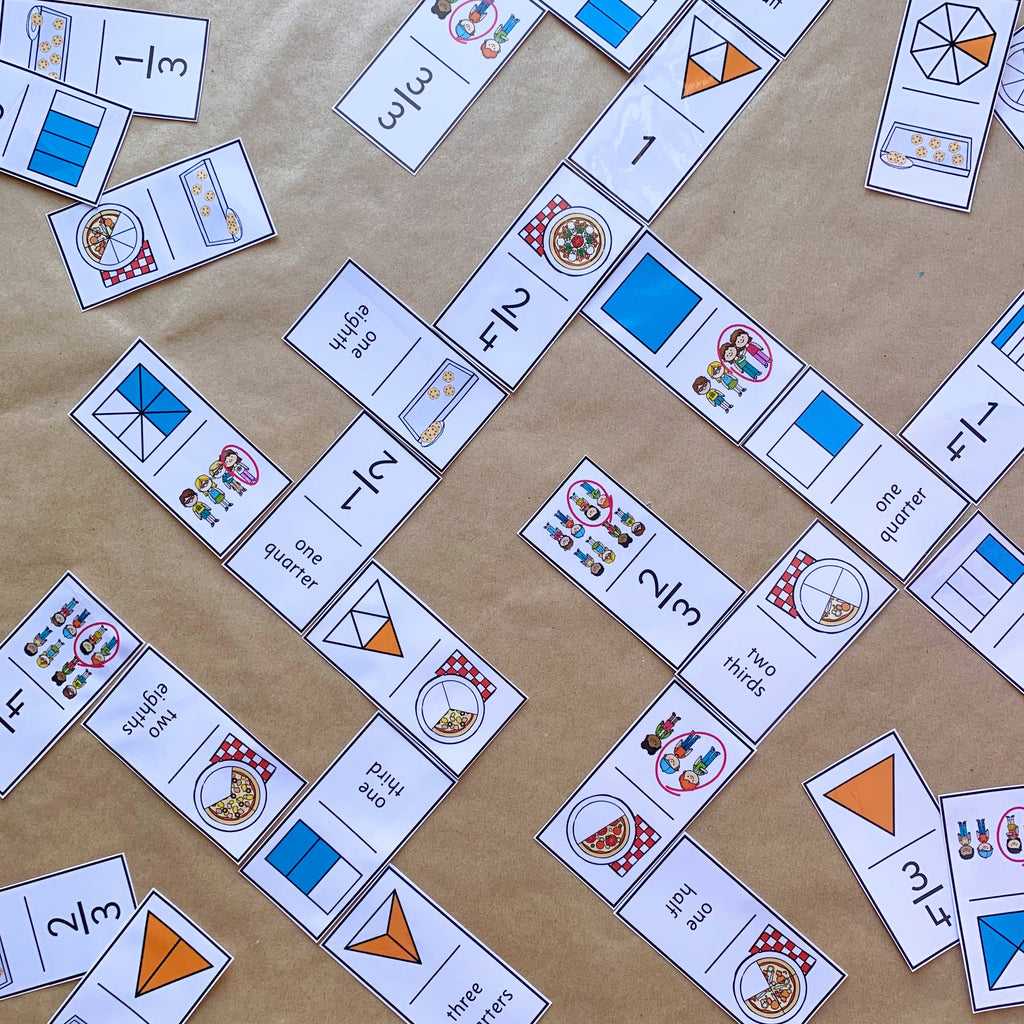# Fraction Domino Game

\$3.00
Learning about fractions and looking for a fun way to learn to recognize the different fractions?

Try this game of dominoes featuring fractions represented as words, shapes, fractions as food (whole + shared) and groups of people.

2 Different games included! Fractions included – whole, half, quarters, thirds and eighths.
Here's what's included -

• 2 different sets of domino cards - one using only fractions represented as parts of shapes, the other with fractions shown as different representations (written fraction - numerator/denominator, shapes divided into the fraction, pizza fractions, people as a fraction, cookies as a fraction - 10 set of cards per A4 page
• 6 different representations of each fraction (written fraction - numerator/denominator, rectangular shape divided into the fraction, pizza fractions, people as a fraction, alternative shape divided into the fraction, cookies as a fraction - 2 set of cards per A4 page
To use -

You will need to have an up to date version of a PDF Reader like Adobe Reader installed on your computer to open this resource.

Please note - prices are in US dollars